Next: Conclusions Up: Review of the AVP Previous: Areas of Application

# Different Implementations

As seen in the previous section, quite a few different implementations of the AVP has surfaced since the presentation of the the method by P-89. A brief review of these methods will be subject of this section. The original implementation of Peebles has been considered in detail earlier in this thesis and will therefore not be considered here.

In an attempt to find a more rapid convergence of the AVP when dealing with large systems of particles of, say, a thousand, Gia combined it with a generalization of the Zel'dovich approximation. The orbits of the particles were parameterized by [cf. equation (8) in][]Giawhere D(t) is the linear growth factor, and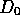is its value at the present epoch. By comparing equation (6.1) to (3.21), one sees that the difference in this implementation is an alternative choice of trial functions. Gia showed that the effect of choosing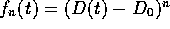is that the convergence of the AVP is faster and that equation (6.1) reproduces the Zel'dovich approximation in the limit of small displacements. The latter reduces the number of possible solutions due to the possibility of avoiding orbit-crossing solutions. By truncating the series in equation (6.1), one retains the search to the quasi-linear regime, where the flow is laminar. Hence, this implementation has faster convergence than Peebles' original implementation, is more accurate, and reduces the multivalued nature of the solutions. The latter is both an advantage and a disadvantage, depending on what sort of systems considered. For systems where small-scale non-linear relative motion is not of interest, the implementation clearly makes the calculations more efficient, while for more complicated systems, the method would ignore possible solutions, such as saddle points.

As an alternative approach to avoid orbit-crossing, and the resulting multivalued solutions, Gia also outlined an implementation where the input data are fields and not particle distributions. This leads to a picture where the density and velocity are determined as a function of fixed comoving Eulerian coordinates. This will put an even greater restriction on the set of possible solutions than in the particle picture, since the velocity is constrained to potential flow. This results in the method not being applicable when having high density contrasts. This field-implementation was discussed further by S-B, which also successfully tested it against one and two dimensional models. Applications to real observational data seemed to them feasible, but computationally costly.

It should also be noted that both Gia and S-B (and later Sch) used an iterative method for locating solutions different from the ones tested in this thesis. A rough outline of the method, which is more of a root finding than an optimizing method, follows: One starts with an initial guess for the coefficients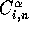and uses them to compute the last term of equation (5.8). Updated values for the coefficients are then computed from the remaining terms, which in turn are used to again compute the last term once again. This process is repeated until convergence.Another implementation of the AVP was presented by SPT-93,SPT-95, applied on large systems of over 500 mass tracers. The present distance is found given the present redshift by the following iterative procedure: The first step is to make an initial guess of the distances to the mass tracers and then solve for the orbits by the method of Steepest Descent.In order to avoid too long commutating time, the termination parameter is set fairly large. As seen in Subsection 5.4.3, the orbit solutions in general are not acceptably close to the observed velocities. The distances are then adjusted by a small amount, and the orbits recalculated. For each tracer, the small change in model redshift is compared to the difference between model and observed redshift, and an estimate is made of the distance shift that will bring the model in line with the observed redshift. The shifting of distance in Subsection 5.4.3 is a rough version of this technique.

P-95 presented a method which can not be considered as just a variation of the AVP, but more of a recreation of the method. Instead of basing the method on Hamilton's principle, it is was now developed using the modified Hamilton's principle [][page 362]Gold. The orbits are in this new version of the AVP parameterized by their positions at a discrete set of world times, opposed to the previous version where the orbits were described as a linear combination of the trial functions (3.21). The resultant action is then given as [cf. equation (8) in][]P-95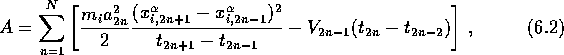whereP-95 used as many as 40 equally spaced time steps from z=80 to the present, i.e. each orbit being described by 120 parameters. This results in a system consisting of 1140 free dimensions for the twelve galaxies considered. The stationary values for the action was determined in the same way as previously, by the use of optimizing methods. Peebles applied the Newton-Raphson method, and due to the impractical inversion of the now very large Hessian matrix, he adjusted the orbits of each galaxy in turn while holding fixed the orbits of the other galaxies. In other words, all the cross terms where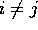in the Hessian were ignored. This technique was mentioned in Subsection 4.3.3.

This version of the AVP, which Peebles also used in his paper from P-96, has a disadvantage over the original version in that the peculiar velocities and the effective mass density contrast at high redshift are poorly defined. But, on the other hand, the computation of the discrete description is considerably faster (e.g. due to no numerical integration) and better adapted for sharply bending orbits. The choice of version is therefore a choice of application.

As seen in the previous section, Sch returned to the idea of using observed redshift to recover distance. They distinguished between two different actions and two different sets of boundary conditions leading to the same equations of motion. The reason for this duality is that they treat the real three-dimensional problem as a combination of two types of coordinates, one is the ``real space'' and the other is the ``redshift space''. The observed redshift is treated in the redshift space, while the other two coordinates is treated in the real space. The two different actions were then used to locate common stationary points by the iterative root finding process outlined in the previous section.Next: Conclusions Up: Review of the AVP Previous: Areas of Application

Trond Hjorteland
Mon Jul 5 02:59:28 MET DST 1999